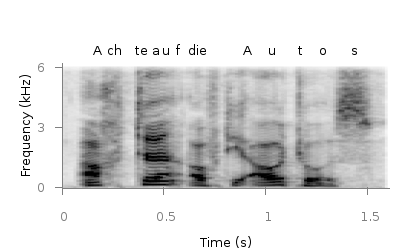# Gnuplotting

Create scientific plots using gnuplot

November 29th, 2011 | 3 Comments

A spectrogram is a time-frequency representation that shows how the spectral content of a signal varies with time. In Fig. 1 the spectrogram of the German sentence “Achte auf die Autos” is shown.Fig. 1 Spectrogram plotted with plot (code to produce this figure, data)

The spectrogram is plotted as mentioned in the color maps entry.

```plot 'spec.dat' binary matrix with image
```

In addition the letters were putted on the graph at their corresponding time of occurrence. The letters itself and their positions are stored in the two lists `syl` and `xpos`. In order to access the single entries of these lists within a for loop the function `word` is needed.

```# Adding the syllables
syl  = 'A    ch   te   a    u    f    d    ie   A    u    t    \
o    s   '
xpos = '0.15 0.22 0.36 0.44 0.49 0.56 0.62 0.66 0.89 1.01 1.16 \
1.26 1.42'
set for [n=1:words(syl)] label word(syl,n) at word(xpos,n),6800
```

Another way to plot the spectrogram is by using `splot` which will result in a different kind of smoothing algorithm of the spectrogram, as can be seen in Fig. 2.Fig. 2 Spectrogram plotted with splot (code to produce this figure, data)

But to get this result we have to fix some of the margins, because `plot` is two-dimensinal and `splot` is three-dimensional which is not desired here.

```set border 10 front ls 11
set tmargin at screen 0.75
set bmargin at screen 0.25
set rmargin at screen 0.95
set lmargin at screen 0.15
```